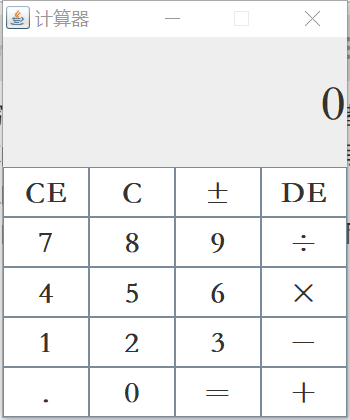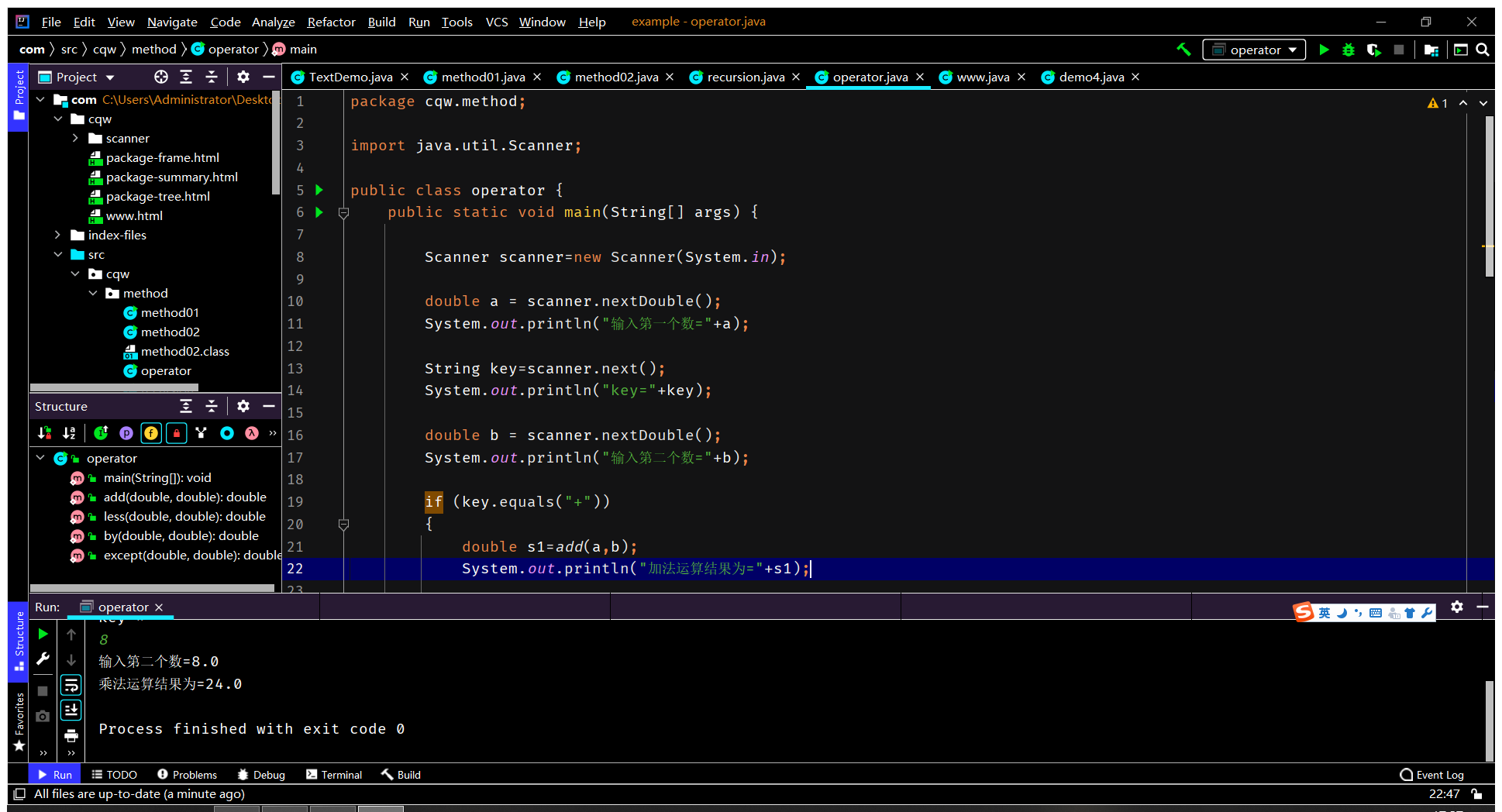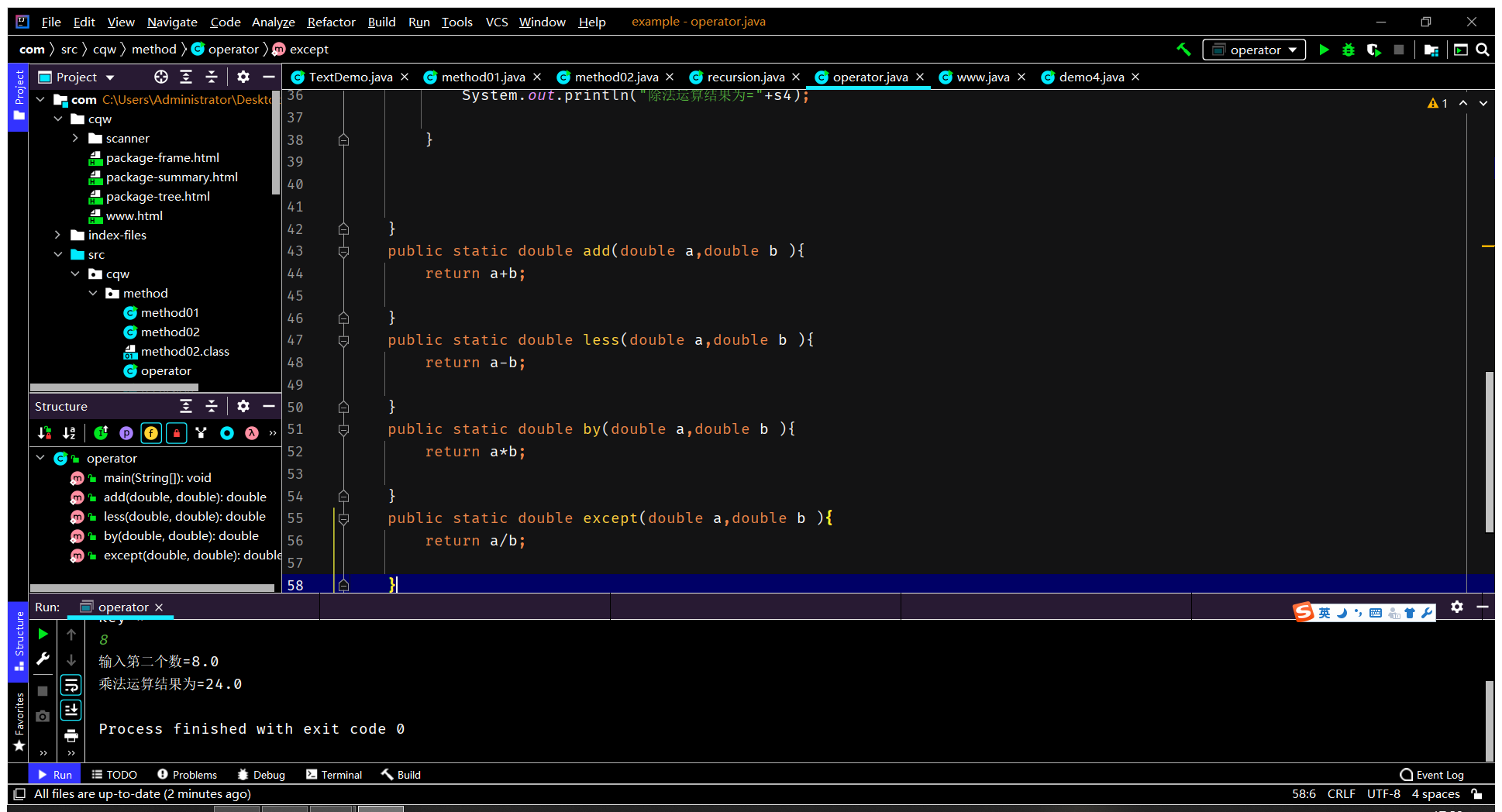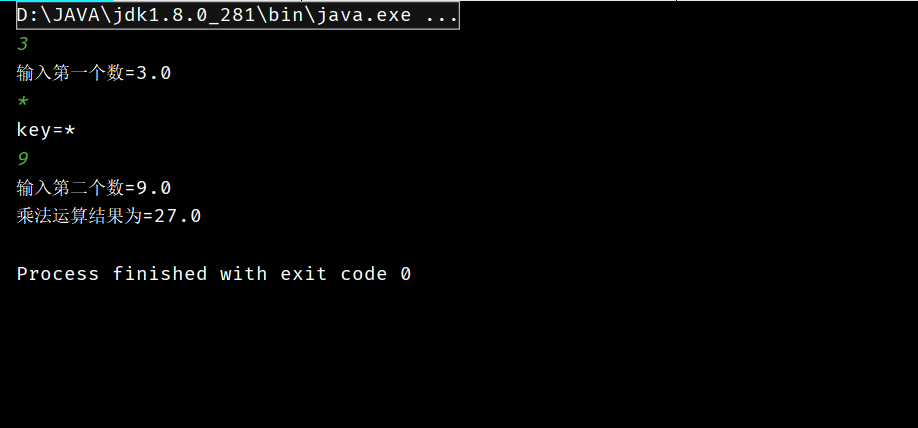• 这是一个用java写的简单计算器，十分适用于java初学者。
• 用java写一个计算器，实现了基本四则运算~~
• 用java实现计算器，实现加减乘除，能够循环接收数据，实现用户交互 思路如下 1、加减乘除的方法 2、利用循环+switch进行用户交互 3、传递需要操作的两数 4、进而输出 代码如下 package com.zxm.method; ...
用java实现计算器，实现加减乘除，能够循环接收数据，实现用户交互
思路如下
1、写四个加减乘除的方法
2、利用循环+switch进行用户交互
3、传递需要操作的两个数
4、进而输出
代码如下
package com.zxm.method;

import java.util.Scanner;
public class Demo7 {
//实现简易计算器
public static void main(String[] args) {
while (true) {
Scanner sc = new Scanner(System.in);
System.out.println("请输入要操作的选项：1 加法 2 减法 3 乘法 4 除法");
String str = sc.next();
char select=str.charAt(0);
switch (select) {
case '1':
System.out.println("请输入第一个数：");
double a = sc.nextDouble();
System.out.println("请输入第二个数：");
double b = sc.nextDouble();
System.out.println("两个数的和为:" + sum);
break;
case '2':
System.out.println("请输入被减数：");
double c = sc.nextDouble();
System.out.println("请输入减数：");
double d = sc.nextDouble();
double difference = subtract(c, d);
System.out.println("两个数的差为:" + difference);
break;
case '3':
System.out.println("请输入第一个数：");
double e = sc.nextDouble();
System.out.println("请输入第二个数：");
double f = sc.nextDouble();
double ride = multiply(e, f);
System.out.println("两个数的积为:" + ride);
break;
case '4':
System.out.println("请输入被除数：");
double g = sc.nextDouble();
System.out.println("请输入除数：");
double h = sc.nextDouble();
double except = divide(g, h);
System.out.println("两个数的商为:" + except);
break;
default:
System.out.println("你的输入有误，请重新输入：");
continue;
}
System.out.println("是否继续？是请输入Y，输入其他退出");
String input = sc.next();
char go = input.charAt(0);
if (go != 'Y'){
break;
}
}
}
public static double add(double a,double b){
return a + b;
}
public static double subtract(double a,double b){
return a - b;
}
public static double multiply(double a,double b){
return a * b;
}
public static double divide(double a,double b){
return a / b;
}
}

运行结果如下
请输入要操作的选项：1 加法 2 减法 3 乘法 4 除法
1
请输入第一个数：
10
请输入第二个数：
5
两个数的和为:15.0
是否继续？是请输入Y，输入其他退出
Y
请输入要操作的选项：1 加法 2 减法 3 乘法 4 除法
2
请输入被减数：
10
请输入减数：
5
两个数的差为:5.0
是否继续？是请输入Y，输入其他退出
Y
请输入要操作的选项：1 加法 2 减法 3 乘法 4 除法
3
请输入第一个数：
10
请输入第二个数：
5
两个数的积为:50.0
是否继续？是请输入Y，输入其他退出
Y
请输入要操作的选项：1 加法 2 减法 3 乘法 4 除法
4
请输入被除数：
10
请输入除数：
5
两个数的商为:2.0
是否继续？是请输入Y，输入其他退出


展开全文• java做的计算器，有两模式：科学计算器，简单计算器
• 用Java编译一个计算器 可以基本上实现+-*/的基本功能 可以供学习Java学习
• 用Java写的简单计算器，可以实现计算器的简单计算
• 用Java写的简易计算器，结构很清晰明了，适合初学者学习
• 用一个Java编写一个计算器程序，实现double数据类型的加减乘除
• 六安职业技术学院毕业 设计论文 用JAVA一个计算器 姓 名 古 松 泽 学 号 20103113 班 级 软件技术1002 指 导 教 师 胡 君 所 在 系 部 信息工程系 二一三年六月 毕业论文设计开题报告 学生姓名 古松泽 班级 软件...
• 用java设计一个计算器 毕业论文 毕业设计论文 用JAVA一个计算器 姓 名 学 号 班 级 软件技术1002 指 导 教 师 所 在 系 部 信息工程系 二一三年六月 毕业论文设计开题报告 学生姓名 班级 软件技术1002 指导教师 ...
• java写的简易计算器，辛苦了两天才完的，整体来说还比较好的
• 用java写的简单计算器程序，模仿windows自带的计算器简单型，采用了布局管理器。
• java写的Android计算器，支持四则运算，支持括号，支持混合运算，支持小数，正数，负数。
• 笔者今天要对用Java写计算器的过程进行一些总结。笔者认为，计算器的核心部分就是加减乘除运算的代码，这也是笔者这小白在代码过程中遇到的困难。笔者通过在博客上的查询和学习，解决了这问题。 思路：运算时...
笔者今天要对用Java写计算器的过程进行一些总结。笔者认为，计算器的核心部分就是加减乘除运算的代码，这也是笔者这个小白在写代码过程中遇到的困难。笔者通过在博客上的查询和学习，解决了这个问题。 思路：运算时通过递归的思想得出结果。截图和代码如下。import java.awt.*;
import java.awt.event.*;
import javax.swing.*;

class Calculate extends JFrame implements ActionListener {
// 定义窗口所需组件
private Container c = getContentPane();
private JPanel p = new JPanel();
private JButton[] jbt = new JButton;
private JLabel jla1 = new JLabel("", SwingConstants.RIGHT);// 右对齐
private JLabel jla2 = new JLabel("0", SwingConstants.RIGHT);// 右对齐
private boolean total;// 定义total来检测小数点是否能加小数点
private String str1 = "", str2 = "";// 定义两个字符串
// 构造方法
Calculate() {
super("计算器");
// 设置布局管理器
p.setLayout(new GridLayout(5, 4));
// 用for循环创建出0~9这10个按钮的对象
for (int i = 0; i < 10; i++) {
jbt[i] = new JButton(String.valueOf(i));
}
// 创建另外14个按钮的对象
jbt = new JButton(".");
jbt = new JButton("＋");
jbt = new JButton("－");
jbt = new JButton("×");
jbt = new JButton("÷");
jbt = new JButton("＝");
jbt = new JButton("%");
jbt = new JButton("√");
jbt = new JButton("x2");
jbt = new JButton("1/x");
jbt = new JButton("CE");
jbt = new JButton("C");
jbt = new JButton("±");
jbt = new JButton("DE");
// 给按钮注册监听器
for (int i = 0; i < 24; i++)
// 用for设置计算器按钮的样式
for (int i = 0; i < 24; i++) {
jbt[i].setPreferredSize(new Dimension(50, 50));
jbt[i].setFont(new Font("华文仿宋", 1, 30));
jbt[i].setBackground(Color.white);
}
// 设置标签内容样式
jla1.setFont(new Font("华文仿宋", 1, 30));
jla2.setFont(new Font("华文仿宋", 1, 50));
// 将计算器的24个按钮加入中间容器p中
// 向容器按规定布局管理器添加组件

setBounds(200, 200, 350, 420);// 设置窗口位置和大小
setVisible(true);// 设置窗口可视化
setResizable(false);// 设置窗口不可改变
setDefaultCloseOperation(JFrame.EXIT_ON_CLOSE);
}
// 实现接口中的方法
public void actionPerformed(ActionEvent e) {
// 实现输入数字按钮功能
if ((JButton) e.getSource() == jbt || (JButton) e.getSource() == jbt || (JButton) e.getSource() == jbt
|| (JButton) e.getSource() == jbt || (JButton) e.getSource() == jbt
|| (JButton) e.getSource() == jbt || (JButton) e.getSource() == jbt
|| (JButton) e.getSource() == jbt || (JButton) e.getSource() == jbt
|| (JButton) e.getSource() == jbt || (JButton) e.getSource() == jbt
|| (JButton) e.getSource() == jbt) {
NumberButton(e);
}
// 实现输入符号按钮功能
if ((JButton) e.getSource() == jbt || (JButton) e.getSource() == jbt
|| (JButton) e.getSource() == jbt || (JButton) e.getSource() == jbt) {
CalculaterButton(e);
}
// 实现=按钮功能
if (e.getActionCommand().equals("＝"))
Calculater();
// 实现C按钮功能
if (e.getActionCommand().equals("C"))
CButton(e);
// 实现CE按钮功能
if (e.getActionCommand().equals("CE"))
CEButton(e);
// 实现DE按钮功能
if (e.getActionCommand().equals("DE"))
DEButton(e);
}// end actionPerformed

数字和符号输入按钮的实现方法
// 定义数字输入方法
private void NumberButton(ActionEvent e) {
// 小数点按钮的功能（判断）小数点是否只出现一次
if (e.getActionCommand().equals(".") && (total == false)) {
if (jla2.getText() == "0") {
str2 = "0.";
jla2.setText(str2);
total = true;
} else {
str2 += e.getActionCommand();
jla2.setText(str2);
total = true;
}
}
// 按0按钮
if (e.getActionCommand().equals("0")) {
// 如果jla2为0
if (jla2.getText().equals("0")) {
str2 = "0";
jla2.setText(str2);
} else {
str2 += e.getActionCommand();
jla2.setText(str2);
}
}
// 1~9这9个按钮的功能
if ((JButton) e.getSource() == jbt || (JButton) e.getSource() == jbt || (JButton) e.getSource() == jbt
|| (JButton) e.getSource() == jbt || (JButton) e.getSource() == jbt
|| (JButton) e.getSource() == jbt || (JButton) e.getSource() == jbt
|| (JButton) e.getSource() == jbt || (JButton) e.getSource() == jbt) {
if (jla2.getText().equals("0")) {
str2 = e.getActionCommand();
jla2.setText(str2);
} else {
str2 += e.getActionCommand();
jla2.setText(str2);
}
}
// ±按钮的功能
if (e.getActionCommand().equals("±")) {
// 正数变负数
if ((java.lang.Double.valueOf(jla2.getText())) > 0)
jla2.setText("-" + jla2.getText());
// 负数变正数
else if ((java.lang.Double.valueOf(jla2.getText())) < 0)
jla2.setText(jla2.getText().replace("-", ""));
}
}// end NumberButton
// 定义运算符号的实现方法
private void CalculaterButton(ActionEvent e) {
str1 += jla2.getText() + e.getActionCommand();
jla1.setText(str1);
jla2.setText("0");
total = false;
}// end CalculaterButton
// =按钮的功能实现方法
private void Calculater() {
jla1.setText("");
str1 += jla2.getText();
double result = parse(str1);
jla1.setText(str1 + "＝");
jla2.setText(Double.valueOf(result) + "");
str1 = str2 = "";
}// end Calculater


计算方法（递归思想）：把运算符号前的数一个一个取出来
private double parse(String str1) {
int index = str1.indexOf("＋");
if (index != -1)
return parse(str1.substring(0, index)) + parse(str1.substring(index + 1));
index = str1.lastIndexOf("－");
if (index != -1)
return parse(str1.substring(0, index)) - parse(str1.substring(index + 1));
index = str1.indexOf("×");
if (index != -1) {
return parse(str1.substring(0, index)) * parse(str1.substring(index + 1));
}
index = str1.indexOf("÷");
if (index != -1) {
return parse(str1.substring(0, index)) / parse(str1.substring(index + 1));
}
return Double.parseDouble(str1);
}

其他键功能
// DE按钮的功能实现方法
private void DEButton(ActionEvent e) {
if (jla2.getText().length() == 1) {
str2 = "0";
jla2.setText(str2);
} else {
str2 = DeteleString(jla2.getText());
jla2.setText(str2);
}
}// end DEButton

// 删除方法
private String DeteleString(String text) {
String a = "";
char[] ch = jla2.getText().toCharArray();
for (int i = 0; i < jla2.getText().length() - 1; i++)
a += ch[i];
if (a.contains("."))
total = true;
else
total = false;
return a;
}// end DeteleString

// CE按钮的功能实现方法
private void CEButton(ActionEvent e) {
str2 = "0";
jla2.setText(str2);
total = false;
}// end CEButton

// C按钮的功能实现方法
private void CButton(ActionEvent e) {
str2 = "0";
str1 = "";
total = false;
jla1.setText(str1);
jla2.setText(str2);
}// end CButton
}// end Calculate

主方法
public class CalculateDemo {
public static void main(String[] args) {
new Calculate();
}// end main
}// end CalculateDemo

public void setPreferredSize(Dimension preferredSize)此方法用于设置此组件的首选大小。如果 preferredSize 为 null，则要求 UI 提供首选大小。 Dimension(int width, int height) 此构造方法用于构造一个 Dimension，并将其初始化为指定宽度和高度（可为负数）。
总结：以上便是笔者对写代码过程的总结，其中的不足就是16~19这四个按钮功能未给予实现方法。另外，笔者也十分欢迎读者对笔者提出一些建议或者代码中的不足。
展开全文• 如何用java写一个简易的计算器界面 前言（与本节重点无关可以忽视） 最近了解了一些界面窗口知识，在老师一步步的悉心教导下我却又一次地走向了迷茫——陌生，理解不充分，照搬。咳咳，但是经过几天的拆解，琢磨，...
如何用java写一个简易的计算器界面
前言（与本节重点无关可以忽视）
最近了解了一些界面窗口知识，在老师一步步的悉心教导下我却又一次地走向了迷茫——陌生，理解不充分，照搬。咳咳，但是经过几天的拆解，琢磨，实践，我也渐渐粗浅地认识到了一些本质问题。当时教写的是登录界面，所以这次我打算写一个计算器界面来检测一下自己是不是依然一头雾水。
基本准备
首先给类取名CaculatorInterface(计算器界面)
package kallen1014;

public class CalculatorInterface {

}

第二步，想好需要引入的包，框架JFrame，按键JButton,输入框JTextFiled，其尺寸Dimension，然后是排版FlowLayout，还有按键的颜色（实际上不要也罢，但在这里还是体现一下这个功能吧）
package kallen1014;

import java.awt.FlowLayout;
import java.awt.Dimension;
import java.awt.Color;
import javax.swing.JFrame;
import javax.swing.JTextField;
import javax.swing.JButton;
public class CalculatorInterface {

}

调用主函数创建对象，并令其实现方法
public static void main(String[]args) {
CalculatorInterface ci=new CalculatorInterface();
ci.init();

}

在方法“init”中编些界面的主要内容
创建框架，包括其格式，标题，显示位置等等
public void init() {
JFrame jf=new JFrame();
jf.setTitle("计算器");
jf.setSize(500, 400);
jf.setResizable(true);//因为不知道需要多大这里的大小设置为可调式
jf.setLocation(null);//程序弹出的位置设置在屏幕中间
jf.setDefaultCloseOperation(JFrame.EXIT_ON_CLOSE);//不设置这个程序将无法关闭

设置布局规则
这一步很重要，决定了一下设置元素的排版，我们这里使用流式布局器让所有元素按照顺序排列，或许你会觉得下面的顺序就要严格按照顺序来了，其实不然，在最后会有一个添加元素的程序操作，那才是决定排版因素的程序
//设置布局规则
FlowLayout fl=new FlowLayout();
jf.setLayout(fl);

设置文本框（即输入框）
//设置输入框
JTextField inputbox=new JTextField();
//设置框架大小
Dimension dim=new Dimension(400,50);//这个大小设置也是暂定的
//这里设置的大小还未应用到输入框里，所以还要引入,用setPreferredSize来表示
inputbox.setPreferredSize(dim);

	注意，每一个框架内的元素最后都要用程序添加到框架中，否则将只存在一个框架，这一步将在最后进行。

设置按键
由于计算器按键很多所以要在设置前先列出来在这里暂且借用一下系统计算器的排版，由于是简易版，我也只会取部分来编写
//设置按钮
JButton buttn1=new JButton(" % ");
JButton buttn2=new JButton(" CE");
JButton buttn3=new JButton(" C ");
JButton buttn4=new JButton(" ← ");
JButton buttn5=new JButton(" 7 ");
JButton buttn6=new JButton(" 8 ");
JButton buttn7=new JButton(" 9 ");
JButton buttn8=new JButton(" ÷ ");
JButton buttn9=new JButton(" 4 ");
JButton buttn10=new JButton(" 5 ");
JButton buttn11=new JButton(" 6 ");
JButton buttn12=new JButton(" × ");
JButton buttn13=new JButton(" 1 ");
JButton buttn14=new JButton(" 2 ");
JButton buttn15=new JButton(" 3 ");
JButton buttn16=new JButton(" - ");
JButton buttn17=new JButton("帮助");
JButton buttn18=new JButton(" 0 ");
JButton buttn19=new JButton(" ½ ");
JButton buttn20=new JButton(" = ");

给按键设置一个颜色
Color color=new Color(255,255,255);//表示白色
Color color2=new Color(177,183,214);//淡蓝色
buttn1.setBackground(color);
buttn2.setBackground(color);
buttn3.setBackground(color);
...

由于有20 个按键这里就不一一例举了
将元素添加到框架中
在这一步当中计算器的界面就基本实现了，剩下的只需要将元素加到框架中即可，注意，这里的添加顺序决定了按键及框架的排版。
这里我们先试试整体效果，在这之后再添加监听器功能。
//将元素加到框架中
······

设置元素可显示
jf.setVisible(true);//关键操作，没有则不能显示。

让我们看看初步成果显然大小调试不当，那么我们来调一调大小通过测试是257和294，然后我们再调整之前设定的大小，给定数值，固定大小即可
小结
做出来的这个计算器只是个界面，实际功能是无法使用的，下一次的文章中我将实现监听器功能，即点击按钮即可打入输入框，以及点击帮助后弹出第二个界面。那么这次的介绍就到这里，下次再见。
展开全文• 自己计算器，支持表达式求值，开根号，三角函数
• 用java写一个简易计算机
用java写一个简易计算机展开全文• 这是个用java写的小计算器,望大家提出宝贵意见。
• 自己的简单的JAVA计算器，可执行基本运算功能，界面也不错。
• 用java写一个简单的计算器，包含源码、素材、说明 用java写一个简单的计算器，包含源码、素材、说明 用java写一个简单的计算器，包含源码、素材、说明 用java写一个简单的计算器，包含源码、素材、说明
• java写的简单计算器代码 , 实现了加减乘除,开方,小数运算等基本运算
• ## java写一个简单的计算器

万次阅读 多人点赞 2016-10-04 18:19:06
请点击好的，看完介绍，咱们就根据这个杭电oj上1237 这道题，改写一个用GUI出来的 小计算器，类似于这样： 然后 通过输入 显示结果，比如说： 可以看得出来，咱们得到的结果是正确的： 代码： package...
• 共给出5不同类型的计算器，个别的其中可能有一些英汉标点符号小错误，相信懂得JAVA的人是会修改的。
• Java写计算器，很好玩，Java写计算器，很好玩，下载试玩...

# 如何用java写一个计算器java 订阅